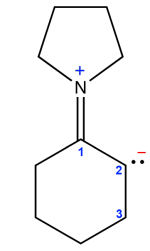Search:

# Detailed study of mesomerism

## (2) Allowed and forbidden Lewis structures

### The limit octet principle

The atoms of non-metals of the first two periods of the periodic table $( H, C, N, O, F)$ are the most frequent in the structural formulas. Their small size does not leave much room around them.

In a structural formula the number of electrons surrounding an atom of the first or second period shall not exceed $8$

### An example of a permitted structureHere there are counted all the electrons surrounding the atom, $2\;e^-$ for each doublet own and another $2\;e^-$ for each covalent bond: - The $N$ atom is surrounded by $8\; e^-$ (4 times $2\; e^-$ for covalent bonds, the formal charge indicates that there is no doublet: permitted. - The $C$ atom number 2 is surrounded by $8 e^-$. As this atom has a formal negative charge, it must be bound to one single $H$ atom, so it is surrounded by the 6 $e^-$ of 3 covalent bonds and 2 electrons from one doublet, so permitted. - The $C$ atom number 3 is surrounded by $8 \;e^-$ (those from two $C-C$ covalent bonds and those of 2 $C-H$ covalent bonds implicitly present in the stylized form), so permitted. Result: Structure permitted

### An example of a not permitted structure- The $N$ atom is surrounded by $10 \;e^-$ (4 times $2 \;e^-$ for covalent bonds and $2\;e^-$ for one doublet, therefore forbidden (though the formal charge is correct) . - The $C$ atom number 2 is surrounded by $6\; e^-$. As this atom has a formal positive charge, it must be attached to only one $H$ atom, so it is surronded by $6\; e^-$ from 3 covalent bonds , so permitted. Result: Structure forbidden